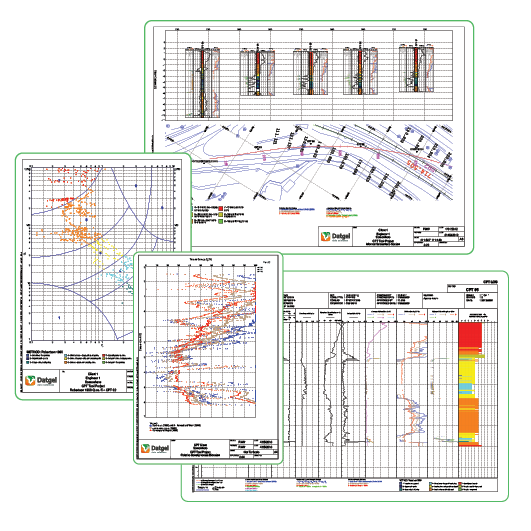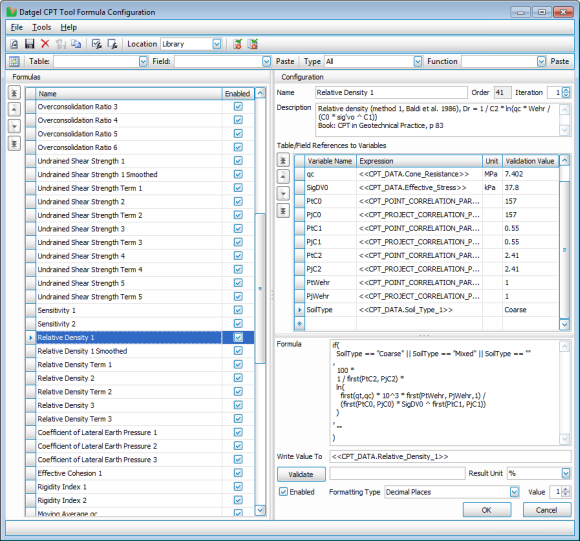# CPT Tool - Correlations# CPT Tool

The most user-definable Cone Penetration Testing software available.

Work without limits as you define which types of soil data to present in log, fence, cross-section and graph reports.

• Define correlation formulas and reports
• 200+ Preconfigured correlations and derivations
• 600+ customisable log, fence/cross-section and graph reports

CPT Tool places advanced reporting directly in the hands of geotechnical engineers with code-free report definition and an array of formula configurations, analysis and report options unmatched in cone penetration testing.

Speed up soil data interpretation with rich-data visualisation in any situation, adapting reporting output to changing requirements and conditions across projects.

# CPT Tool - Correlations

• More than 100 preconfigured correlations included
• Users can edit and create formulas

## Preconfigured correlations• Relative Density Index, Dr
• Undrained shear Strength, su
• SPT N60 and stepped average
• Shear wave velocity, Vs
• Unit Weight, γ
• Small strain shear modulus, G0
• Young's modulus, E
• Constrained Modulus, M
• Compression Index, Cc
• Coefficient of volume, mv
• Preconsolidation stress, σp'
• Over consolidation ratio, OCR
• Friction angle, φ'
• Effective cohesion, c'
• Sensitivity, St
• Coefficient of lateral earth pressure, K0
• Rigidity index, Ir
• Hydraulic conductivity (permeability), K
• State parameter, Ψ
• Fines Content, FC

## User Definable Correlation Formulas

The Datgel Formula Tool is the first way for a user to write a formula in gINT without writing complex VBA code.  Simply define the referenced fields, and write your correlation formula in a standard formula syntax.• Reference any related field in the database
• Built in mathematical and statistical functions
• Define result format, i.e. decimal places or significant figures
• Results may be numbers or string/text
• Store your common formulas in the library, and project specific formulas in the project